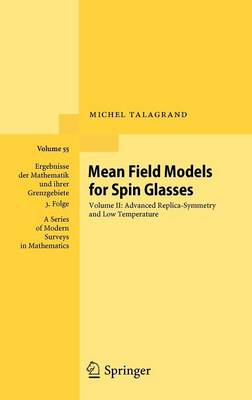•Mean Field Models for Spin Glasses: Volume II: Advanced Replica-Symmetry and Low Temperature - Ergebnisse der Mathematik und ihrer Grenzgebiete. 3. Folge / A Series of Modern Surveys in Mathematics 55 (Hardback)

(author)
£109.99
Hardback 632 Pages / Published: 14/08/2011
• We can order this

Usually dispatched within 3 weeks

This is a new, completely revised, updated and enlarged edition of the author's Ergebnisse vol. 46: "Spin Glasses: A Challenge for Mathematicians" in two volumes (this is the 2nd volume). In the eighties, a group of theoretical physicists introduced several models for certain disordered systems, called "spin glasses". These models are simple and rather canonical random structures, of considerable interest for several branches of science (statistical physics, neural networks and computer science). The physicists studied them by non-rigorous methods and predicted spectacular behaviors. This book introduces in a rigorous manner this exciting new area to the mathematically minded reader. It requires no knowledge whatsoever of any physics. The present Volume II contains a considerable amount of new material, in particular all the fundamental low-temperature results obtained after the publication of the first edition.

Publisher: Springer-Verlag Berlin and Heidelberg GmbH & Co. KG
ISBN: 9783642222528
Number of pages: 632
Weight: 1124 g
Dimensions: 235 x 155 x 34 mm
Edition: 2nd ed. 2011

MEDIA REVIEWS

From the reviews of the second edition:

"This is the second volume of the author's treatise Mean field models for spin glasses ... . With its depth and profusion of important techniques, this book ... opens new perspectives in probability theory and statistical mechanics of disordered systems. It will be a source of inspiration for generations of mathematicians." (Francis Comets, Mathematical Reviews, November, 2013)

"This is the second volume of the treatise `Mean fields models for spin glasses' and it is truly the continuation of the preceding one. ... the book is a book of mathematics expanded around Hamiltonian. The main considered topics are the Gardner formula, the Hopfield model, the SK model, the Parisi formula and the p-spin interaction model. ... This is truly a nice book of condensed mathematics." (Guy Jumarie, Zentralblatt MATH, Vol. 1232, 2012)